Courses
Courses for Kids
Free study material
Offline Centres
More

# NCERT Solutions for Class 9 Maths Chapter 6 - Lines And AnglesLast updated date: 01st Dec 2023
Total views: 777.9k
Views today: 9.77k

## NCERT Solutions for Class 9 Maths Chapter 6 Lines and Angles - Free PDF

Lines and Angles are discussed in Chapter 6 of CBSE Class 9. The chapter discusses crucial ideas such as the pair of angles, vertically opposite angles, and linear parity. For CBSE Class 9 Chapter 6, Vedantu offers NCERT solutions compiled by subject-matter experts. To ace your preparations, download the NCERT solutions that our professionals have offered. The answers to the NCERT exercise questions are provided in an easy-to-understand format and in a step-by-step fashion. Every single question on the Class 9 curriculum is answered in the NCERT answers pdf.

Watch videos on
NCERT Solutions for Class 9 Maths Chapter 6 - Lines And Angles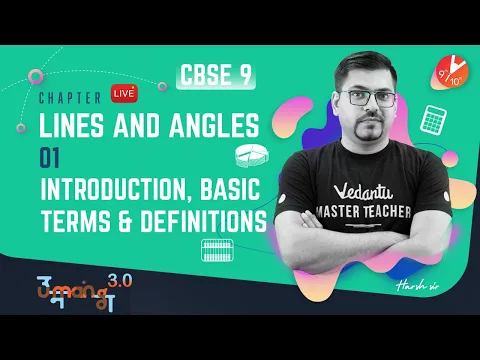LINES AND ANGLES L-1 (Introduction, Basic Terms & Definitions) CBSE 9 Math Chapter 6 | Umang Vedantu
Vedantu 9&10
SubscribeShare
6K likes
139K Views
2 years agoLines and Angles - 1 | NCERT Solutions for Class 9 Maths Chapter 6 | CBSE Board | Vedantu (2019)
Vedantu 9&10
7.1K likes
189.4K Views
4 years ago

## Access NCERT Solutions for Class 9 Maths Chapter 6- Lines and Angles

### Question 1

In the given figure, lines AB and CD intersect at O. if $\angle \text{AOC+}\angle \text{BOE=7}{{\text{0}}^{\text{o}}}$ and $\angle \text{BOD=4}{{\text{0}}^{\text{o}}}$find $\angle \text{BOE}$ and reflex $\angle \text{COE}$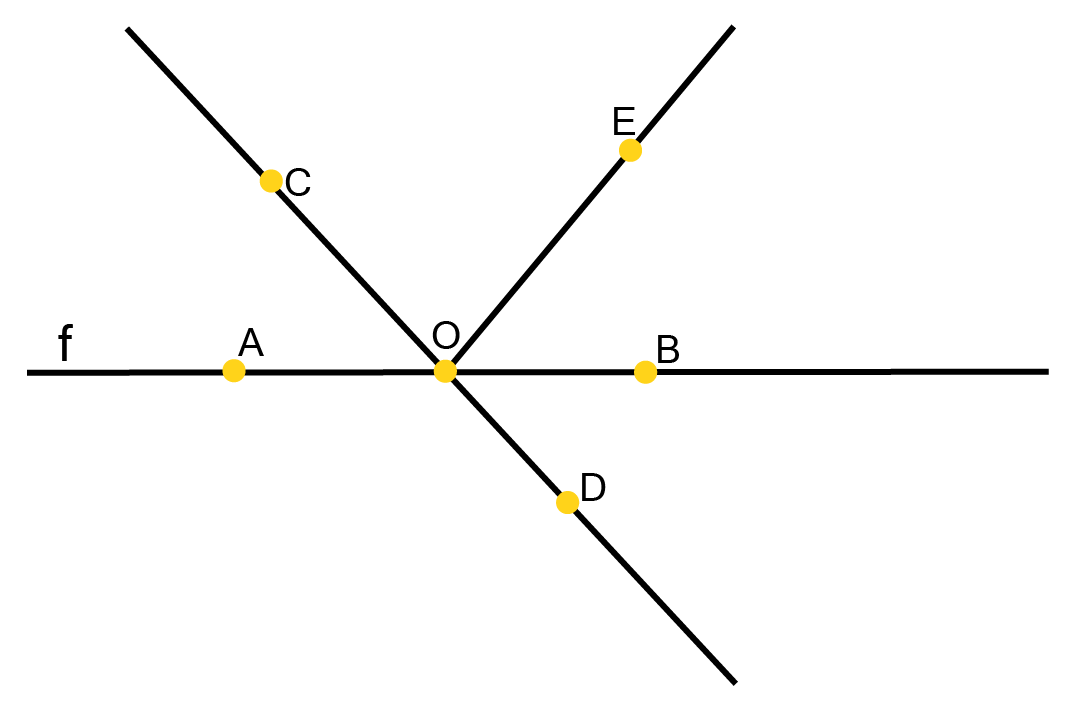Ans:

AB is a straight line, OC and OE are rays from O.

We know that a straight line covers ${{180}^{\circ }}$

$\text{ }\Rightarrow \angle \text{AOC+}\angle \text{COE+}\angle \text{BOE=18}{{\text{0}}^{\circ }}$

By clubbing $\angle \text{AOC and }\angle \text{BOE}$ together we can rewrite the above equation as

$\Rightarrow \left( \angle \text{AOC+}\angle \text{BOE} \right)+\angle \text{COE=18}{{\text{0}}^{\circ }}$

Putting $\text{ }\angle \text{AOC+}\angle \text{BOE=7}{{\text{0}}^{\circ }}$

$\text{ }\Rightarrow \text{7}{{\text{0}}^{\circ }}+\angle \text{COE=18}{{\text{0}}^{\circ }}$

$\text{ }\Rightarrow \angle \text{COE=18}{{\text{0}}^{\circ }}\text{-}{{70}^{\circ }}$

$\text{ }\Rightarrow \angle \text{COE=11}{{\text{0}}^{\circ }}$

Hence  reflex $\text{ }\angle \text{COE=36}{{\text{0}}^{\circ }}\text{-11}{{\text{0}}^{\circ }}$

$\text{ reflex}\angle \text{COE=25}{{\text{0}}^{\circ }}\text{ }$

Similarly CD is a straight line, OB and OE are rays from O.

We know that a straight line covers ${{180}^{\circ }}$

$\text{ }\Rightarrow \angle B\text{OD+}\angle \text{COE+}\angle \text{BOE=18}{{\text{0}}^{\circ }}$

$\text{ }\Rightarrow \text{ }{{40}^{\circ }}\text{+}{{110}^{\circ }}\text{+}\angle \text{BOE=18}{{\text{0}}^{\circ }}$

$\text{ }\Rightarrow \text{ }\angle \text{BOE=18}{{\text{0}}^{\circ }}\text{-}\left( \text{4}{{\text{0}}^{\circ }}\text{+11}{{\text{0}}^{\circ }} \right)$

$\text{ }\Rightarrow \text{ }\angle \text{BOE=18}{{\text{0}}^{\circ }}\text{-}{{150}^{\circ }}$

$\text{ }\Rightarrow \text{ }\angle \text{BOE=}{{30}^{\circ }}$

Hence $\angle \text{BOE=}{{30}^{\circ }}$ and reflex $\angle \text{COE=25}{{\text{0}}^{\circ }}$

### Question 2

In the given figure, lines XY and MN intersect at O. If $\angle \text{POY=9}{{\text{0}}^{\text{o}}}$ and a: b = 2:3, find c.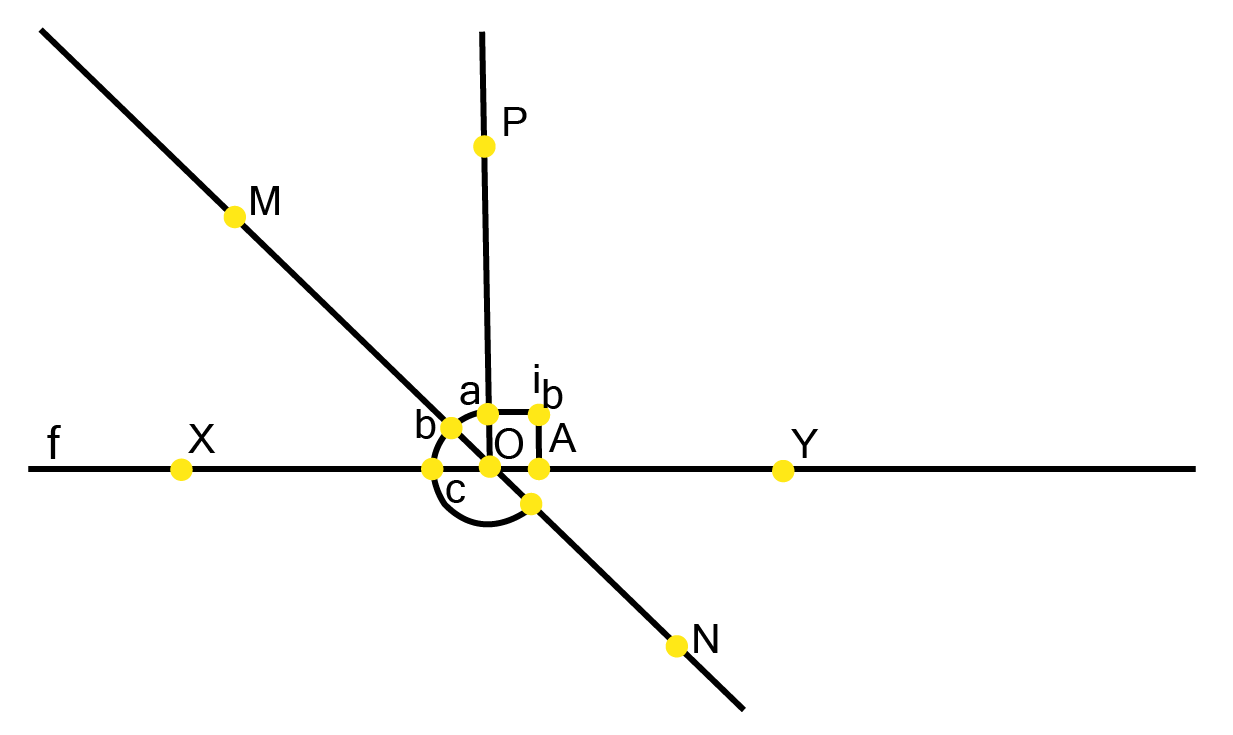Ans:

Let the common ratio between a and b be x.

$\therefore$$\text{a = 2x}$ , and $\text{b = 3x}$

XY is a straight line, OM and OP are rays from O.

We know that a straight line covers ${{180}^{\circ }}$

$\angle \text{XOM +}\angle \text{MOP +}\angle \text{POY = 18}{{\text{0}}^{\text{o}}}$

Putting values for $\angle \text{XOM=b and}\angle \text{MOP=a}$

$\Rightarrow \text{ b + a +}\angle \text{POY = 18}{{\text{0}}^{\text{o}}}$

$\Rightarrow \text{ 3x + 2x +}\angle \text{POY = 18}{{\text{0}}^{\text{o}}}$

$\Rightarrow \text{ 5x = 9}{{\text{0}}^{\text{o}}}$

$\Rightarrow \text{ x = 1}{{\text{8}}^{\text{o}}}$

$\therefore \text{ a = 2x}$

$\Rightarrow \text{ a = 2}\times \text{1}{{\text{8}}^{\circ }}$

$\text{ = 3}{{\text{6}}^{\circ }}$

$\therefore \text{ b = 3x}$

$\Rightarrow \text{ b = 3}\times \text{1}{{\text{8}}^{\circ }}$

$\text{ = 5}{{\text{4}}^{\circ }}$

Similarly MN is a straight line, OX is a ray from O

$\text{ }\therefore \text{b+c=18}{{\text{0}}^{\circ }}$

$\text{5}{{\text{4}}^{\text{o}}}\text{ + c = 18}{{\text{0}}^{\text{o}}}$

$\text{ }c=\text{ }{{180}^{\circ }}\text{ }-\text{ }{{54}^{\circ }}$

$\text{ }c=\text{ 12}{{\text{6}}^{\circ }}$

### Question 3

In the given figure,$\angle \text{PQR=}\angle \text{PRQ}$ , then prove that $\angle \text{PQS=}\angle \text{PRT}$.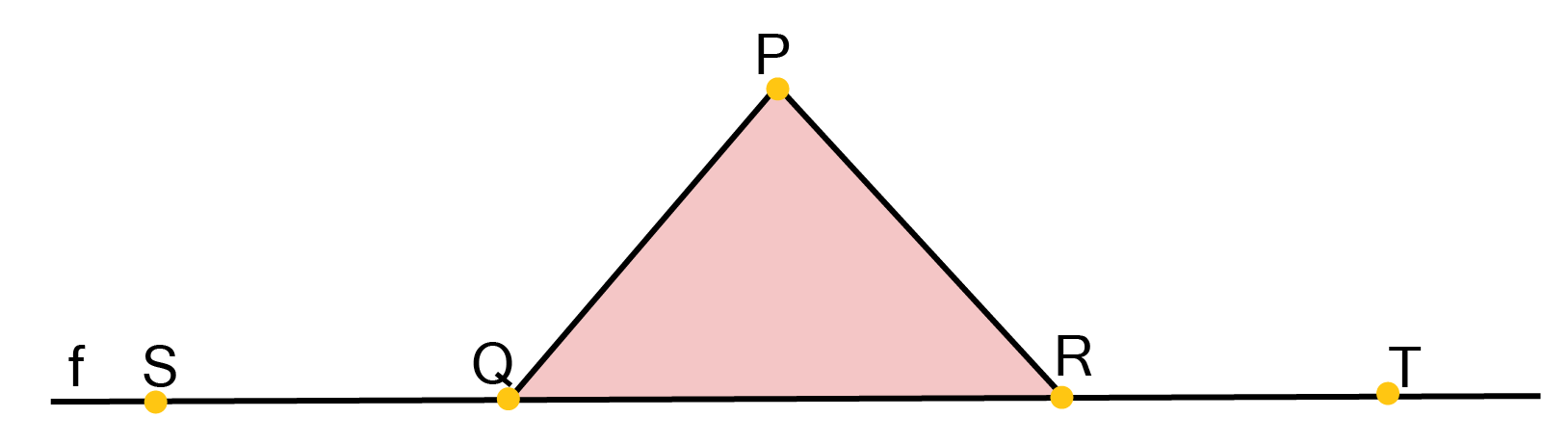Ans:

ST is a straight line, QP is a line segment from Q in ST to any point P

by Linear Pair property

$\angle \text{PQS+}\angle \text{PQR=18}{{\text{0}}^{\text{o}}}$

$\Rightarrow \text{ }\angle \text{PQR=18}{{\text{0}}^{\text{o}}}\text{-}\angle \text{PQS}$ ......($1$ )

Similarly

$\angle PRT+\angle PRQ={{180}^{\circ }}$

$\Rightarrow \text{ }\angle \text{PRQ=18}{{\text{0}}^{\text{o}}}\text{-}\angle \text{PRT}$ ......($2$ )

Now in the question it is given that $\angle \text{PQR=}\angle \text{PRQ}$

Therefore on equating equation ($1$ ) and ($2$ ) we get

$\text{18}{{\text{0}}^{\text{o}}}\text{-}\angle \text{PQS=18}{{\text{0}}^{\text{o}}}\text{-}\angle \text{PRT}$

$\Rightarrow \text{ }\angle \text{PQS=}\angle \text{PRT}$

Hence proved

### Question 4

In the given figure, if $\text{x+y=w+z}$ then prove that AOB is a line.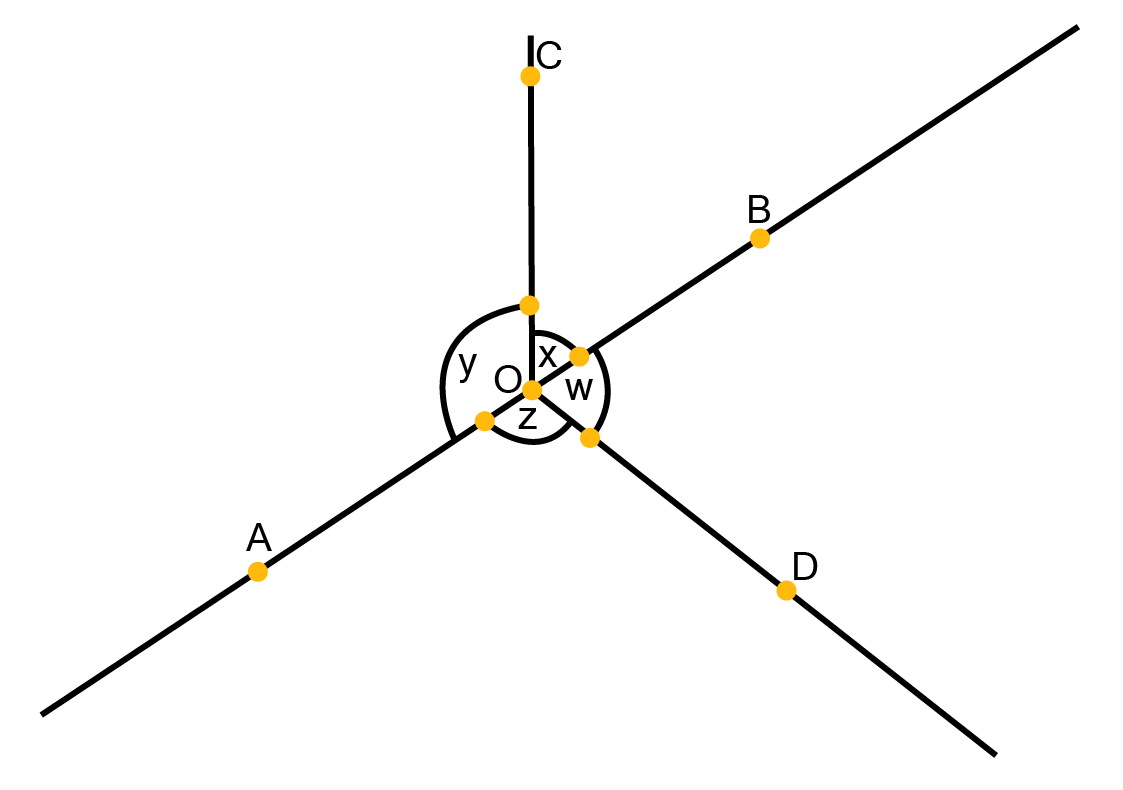Ans:

It can be observed that,

Since there are ${{360}^{\circ }}$ around a point therefore we can write

$x+y+z+w={{360}^{\circ }}$

It is given that,

$\text{x+y=w+z}$

Therefore writing$\text{x+y}$in place of $\text{w+z}$so that we can eliminate w and z, we get

$x+y+x+y={{360}^{\circ }}$

$2\left( x+y \right)={{360}^{\circ }}$

$x+y={{180}^{\circ }}$

Since x and y form a linear pair, hence we can say that AOB is a line.

Hence proved

### Question 5

In the given figure, POQ is a line. Ray OR is perpendicular to line PQ. OS is another ray lying between rays OP and OR. Prove that

$\angle \text{ROS=}\frac{\text{1}}{\text{2}}\left( \angle \text{QOS-}\angle \text{POS} \right)$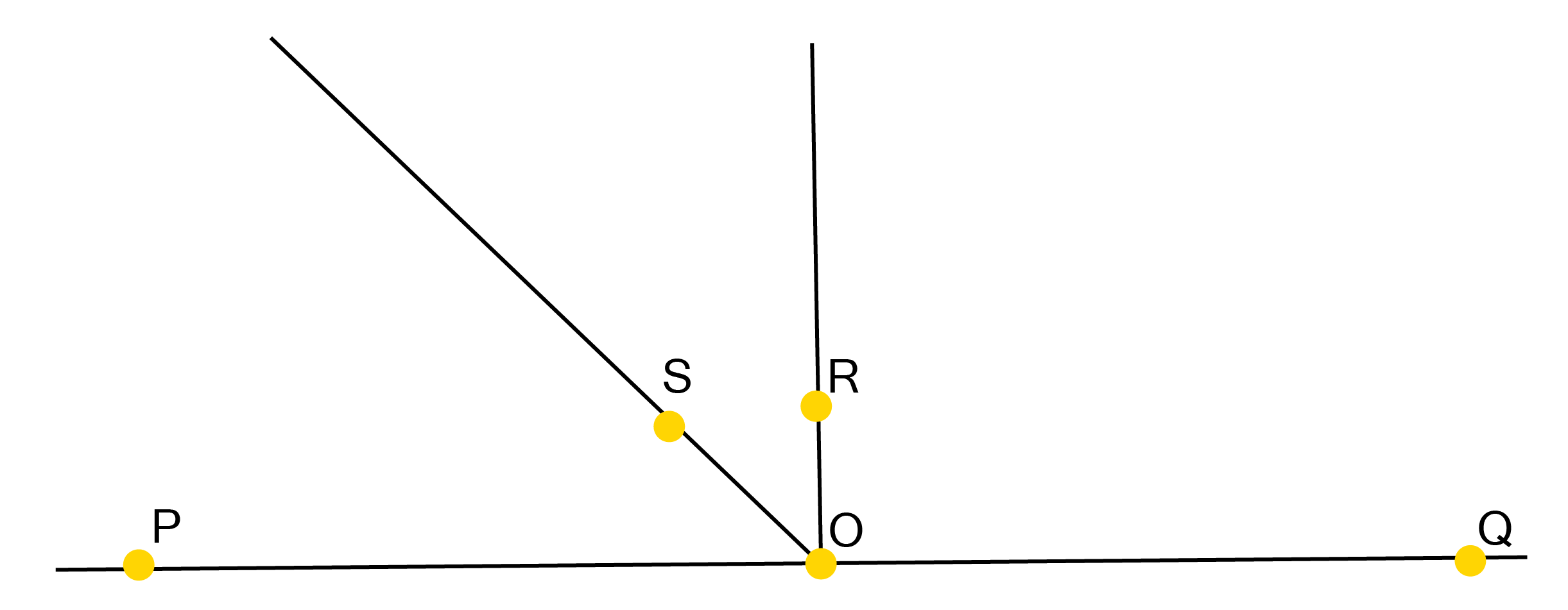Ans:

Since $\text{OR}\bot \text{PQ}$  therefore

$\angle \text{POR=9}{{\text{0}}^{\text{o}}}$

$\angle POS+ROS={{90}^{\circ }}$

$\Rightarrow \text{ }\angle \text{ROS=9}{{\text{0}}^{\text{o}}}\text{-}\angle \text{POS}$ ......   ($1$ )

Similarly $\angle \text{QOR=9}{{\text{0}}^{\text{o}}}$ (Since $\text{OR }\!\!\hat{\ }\!\!\text{ PQ}$)

$\therefore \angle \text{QOS-}\angle \text{ROS=9}{{\text{0}}^{\text{o}}}$

$\Rightarrow \text{ }\angle \text{ROS=}\angle \text{QOS-9}{{\text{0}}^{\text{o}}}$......($2$)

We can clearly see that on adding equation ($1$ ) and ($2$) ${{90}^{\circ }}$ get canceled out

$\Rightarrow \text{2}\angle \text{ROS=}\angle \text{QOS-}\angle \text{POS}$

Which can easily be written as

$\Rightarrow \angle \text{ROS=}\frac{\text{1}}{\text{2}}\left( \angle \text{ROS-}\angle \text{POS} \right)$

Hence proved

### Question 6

It is given that $\text{XYZ=6}{{\text{4}}^{\text{o}}}$ and XY is produced to point P. Draw a figure from the given information. If ray YQ bisects $\text{ZYP}$, find $\text{XYQ}$ and reflex$\text{QYP}$.

Ans:

It is given that line YQ bisects $\angle \text{ZYP}$.

Hence, $\angle \text{QYP=}\angle \text{ZYQ}$

It can easily be understood that PX is a line, YQ and YZ being rays standing on it.

$\angle \text{XYZ+}\angle \text{ZYQ+}\angle \text{QYP=18}{{\text{0}}^{\text{o}}}$

From above relation $\angle \text{QYP=}\angle \text{ZYQ}$ we can write

$\text{6}{{\text{4}}^{\text{o}}}\text{+2}\angle \text{QYP=18}{{\text{0}}^{\text{o}}}$

$\Rightarrow \text{ 2}\angle \text{QYP=18}{{\text{0}}^{\text{o}}}\text{-6}{{\text{4}}^{\text{o}}}$

$\Rightarrow \text{ }\angle \text{QYP=5}{{\text{8}}^{\circ }}$

Therefore $\angle \text{ZYQ=5}{{\text{8}}^{\circ }}$

Also Reflex $\angle \text{QYP=30}{{\text{2}}^{\circ }}$

Now we can write $\angle \text{XYQ}$ as below

$\angle \text{XYQ=}\angle \text{XYZ+}\angle \text{ZYQ}$

${{64}^{\circ }}+{{58}^{\circ }}={{122}^{\circ }}$

Therefore we found $\angle \text{XYQ=12}{{\text{2}}^{\circ }}$ and so the Reflex $\angle \text{QYP=30}{{\text{2}}^{\circ }}$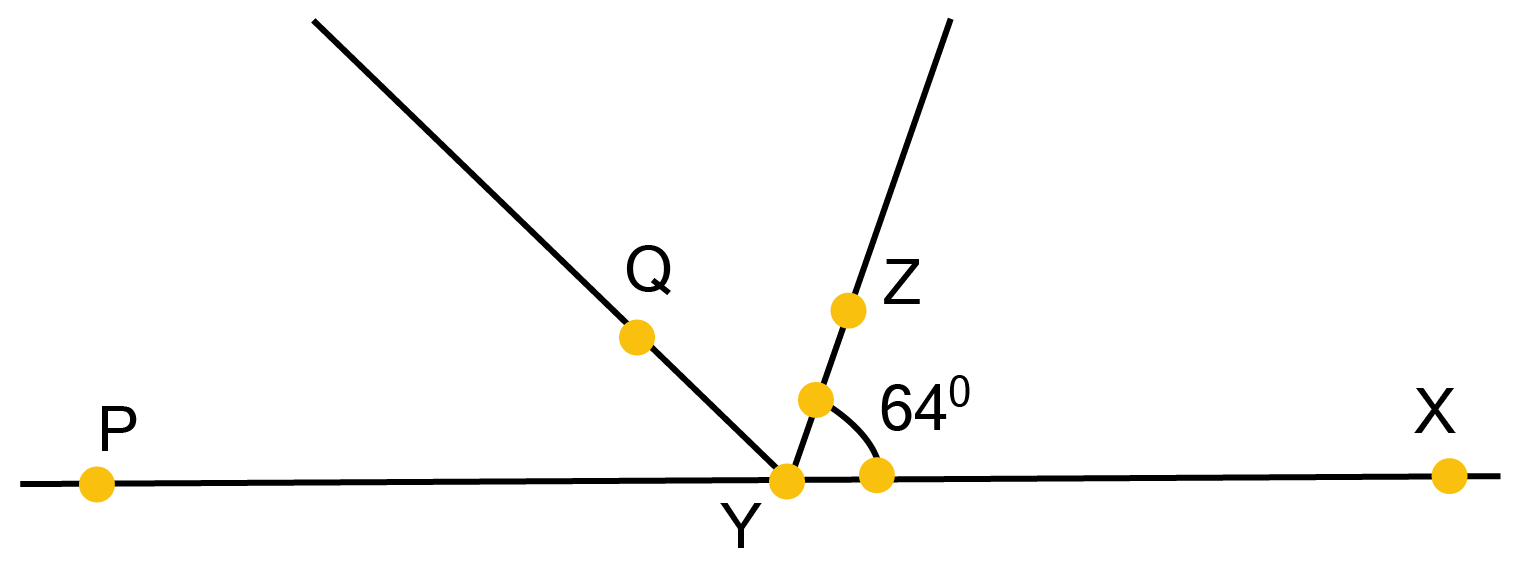### Question 1

In the given figure, find the values of x and y and then show that AB $\parallel$ CD.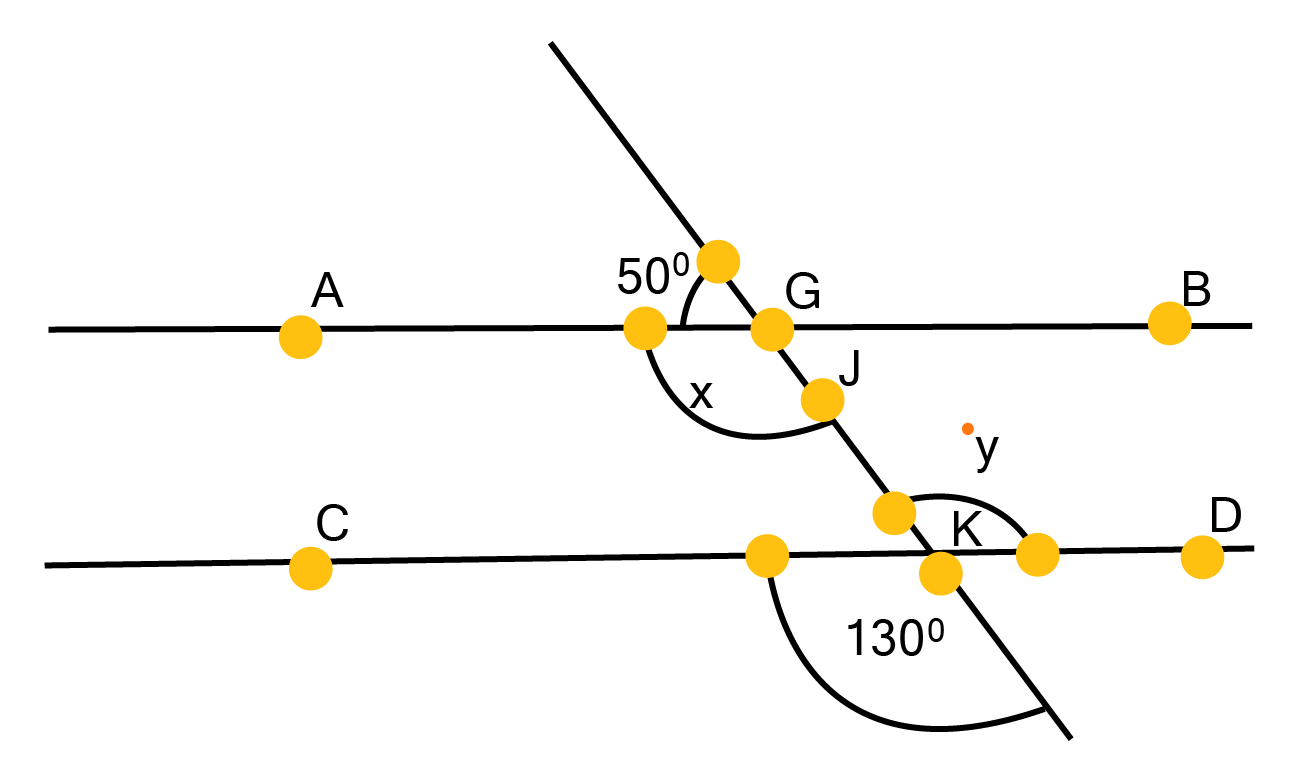Ans:

From the figure it is clear that,

${{50}^{\circ }}+x={{180}^{\circ }}$ (Linear Pair)

$\Rightarrow \text{ x=13}{{\text{0}}^{\text{o}}}$......($1$ )

Also, from the figure it is clear that $y={{130}^{\circ }}$ (since vertically opposite angles are equal)

Similarly we can see that  x and y are alternate interior angles for lines AB and CD and so measures of these angles are equal to each other.

Therefore, line AB || CD.

Hence proved.

### Question 2

In the given figure, if AB $\parallel$ CD, CD $\parallel$ EF and y: z = 3:7, find x.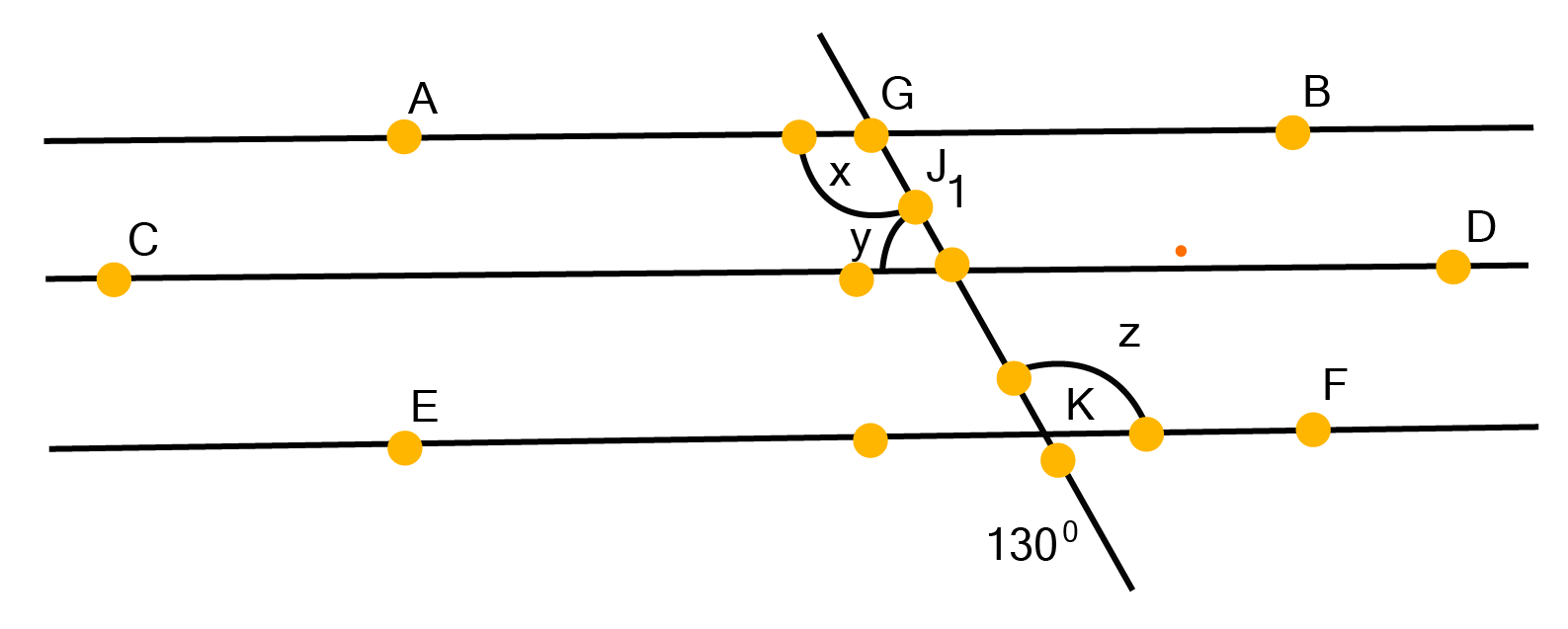Ans:

It is given that AB$\parallel$ CD and CD $\parallel$ EF

$\therefore$ AB$\parallel$CD$\parallel$ EF (Lines parallel to other fixed line are parallel to each other)

It can easily be understood that

$\text{x=z}$  (since alternate interior angles are equal) ...... ($1$)

It is given that y: z = 3: 7

Without any loss of generality we can let $\text{y=3a}$and $\text{z=7a}$

Also, $\text{x+y=18}{{\text{0}}^{\text{o}}}$ (Co-interior angles together sum up to ${{180}^{\circ }}$)

From equation ($1$) we can write z in place of x as shown

$\text{z+y=18}{{\text{0}}^{\text{o}}}$

It can further written as shown

$7a+3a={{180}^{\circ }}$

$\Rightarrow \text{ 10a=18}{{\text{0}}^{\text{o}}}$

$\Rightarrow \text{ a=1}{{\text{8}}^{\text{o}}}$

$\therefore \text{ x=7 }\!\!\times\!\!\text{ 1}{{\text{8}}^{\text{o}}}$

$\therefore \text{ x=12}{{\text{6}}^{\text{o}}}$

### Question 3

In the given figure, If AB$\parallel$CD, EF$\bot$ CD and$\angle \text{GED=12}{{\text{6}}^{\text{o}}}$ , find$\angle \text{AGE}$ , $\angle \text{GEF}$and $\angle \text{FGE}$.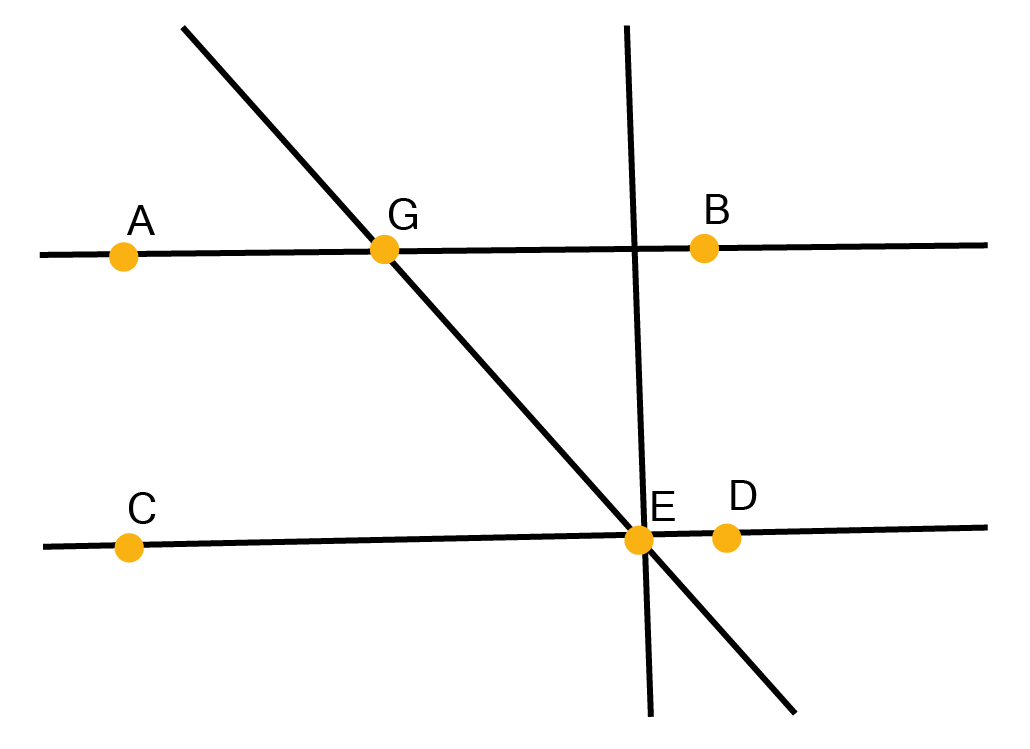Ans:

We are given that,

AB $\parallel$ CD and EF$\bot$ CD and

$\text{ }\angle \text{GED=12}{{\text{6}}^{\text{o}}}$

Which can be written as

$\Rightarrow \angle \text{GEF+}\angle \text{FED=12}{{\text{6}}^{\text{o}}}$

$\Rightarrow \text{ }\angle \text{GEF+9}{{\text{0}}^{\text{o}}}\text{=12}{{\text{6}}^{\text{o}}}$

Hence we can obtain $\text{GEF}$as shown below

$\Rightarrow \text{ }\angle \text{GEF=12}{{\text{6}}^{\text{o}}}\text{-9}{{\text{0}}^{\text{o}}}$

$\Rightarrow \text{ }\angle \text{GEF=3}{{\text{6}}^{\circ }}$

$\angle \text{AGE=}\angle \text{GED=12}{{\text{6}}^{\text{o}}}$ (∠AGE and ∠GED are alternate interior angles)

But

$\angle \text{AGE+}\angle \text{FGE=18}{{\text{0}}^{\text{o}}}$ (because these form a linear pair)

$\Rightarrow \text{12}{{\text{6}}^{\text{o}}}\text{+}\angle \text{FGE=18}{{\text{0}}^{\text{o}}}$

$\Rightarrow \angle \text{FGE=18}{{\text{0}}^{\text{o}}}\text{-12}{{\text{6}}^{\text{o}}}$

$\Rightarrow \angle \text{FGE=5}{{\text{4}}^{\text{o}}}$

Hence, we found $\angle \text{AGE=12}{{\text{6}}^{\text{o}}}$, $\angle \text{GEF=3}{{\text{6}}^{\text{o}}}$ , $\angle \text{FGE=5}{{\text{4}}^{\text{o}}}$

### Question 4

In the given figure, if PQ $\parallel$ ST, $\angle \text{PQR=11}{{\text{0}}^{\text{o}}}$ and$\angle \text{RST=13}{{\text{0}}^{\text{o}}}$ , find $\angle \text{QRS}$.

(Hint: Draw a line parallel to ST through point R.)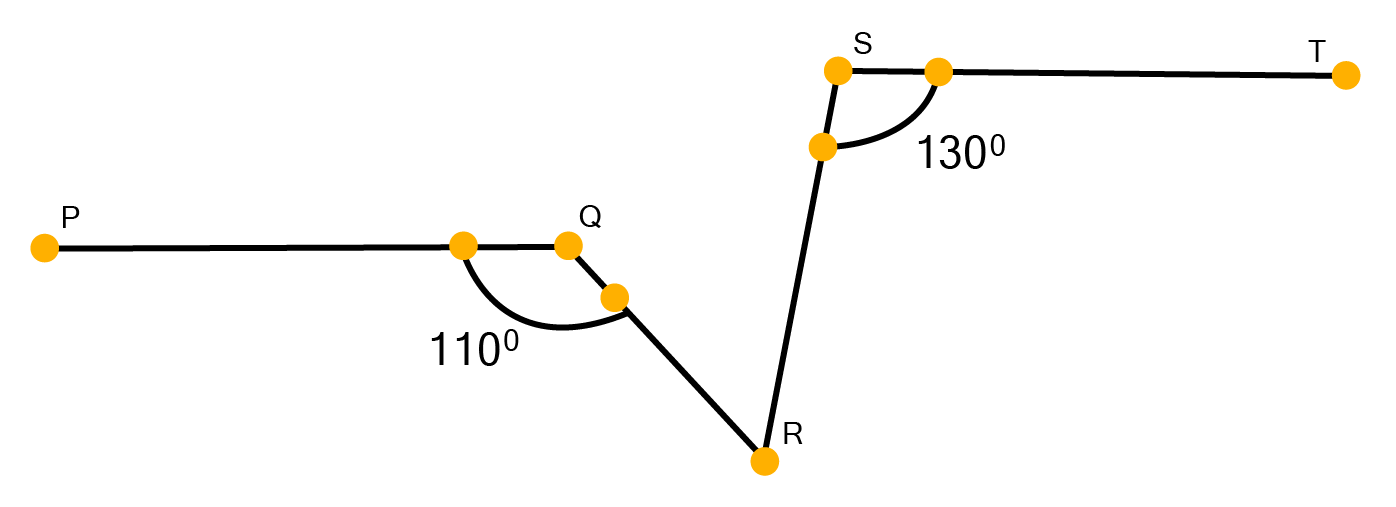Ans: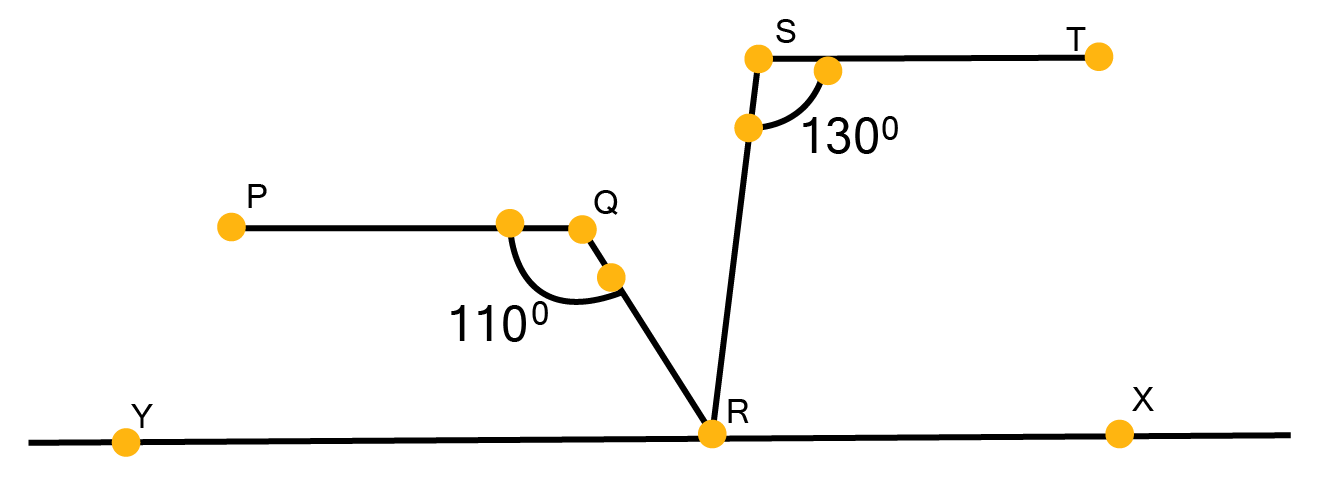In this question we will have some construction of our own, we draw a line XY parallel to ST and so parallel to PQ passing through point R.

$\angle \text{PQR+}\angle \text{QRX=18}{{\text{0}}^{\text{o}}}$ (Co-interior angles on the same side of transversal QR together sum up to ${{180}^{\circ }}$)

$\therefore \text{11}{{\text{0}}^{\text{o}}}\text{+}\angle \text{QRX=18}{{\text{0}}^{\text{o}}}$

$\Rightarrow \text{ }\angle \text{QRX=7}{{\text{0}}^{\text{o}}}$

Also,

$\angle \text{RST+}\angle \text{SRY=18}{{\text{0}}^{\circ }}$  (sum of Co-interior angles on the same side of transversal SR equals $\text{18}{{\text{0}}^{\circ }}$)

$\Rightarrow \text{ }\angle \text{SRY=18}{{\text{0}}^{\circ }}\text{-13}{{\text{0}}^{\circ }}$

$\Rightarrow \text{ }\angle \text{SRY=}{{50}^{\circ }}$

Now from the construction XY is a straight line. RQ and RS are rays from it.

$\angle \text{QRX+}\angle \text{QRS+}\angle \text{SRY=18}{{\text{0}}^{\circ }}$

$\Rightarrow \text{ 7}{{\text{0}}^{\circ }}+\angle QRS+{{50}^{\circ }}={{180}^{\circ }}$

Hence we found that

$\angle \text{QRS=6}{{\text{0}}^{\circ }}$

### Question 5

In the given figure, if AB $\parallel$ CD, $\angle APQ={{50}^{\circ }}$ and$\angle PRD={{127}^{\circ }}$ , find x and y.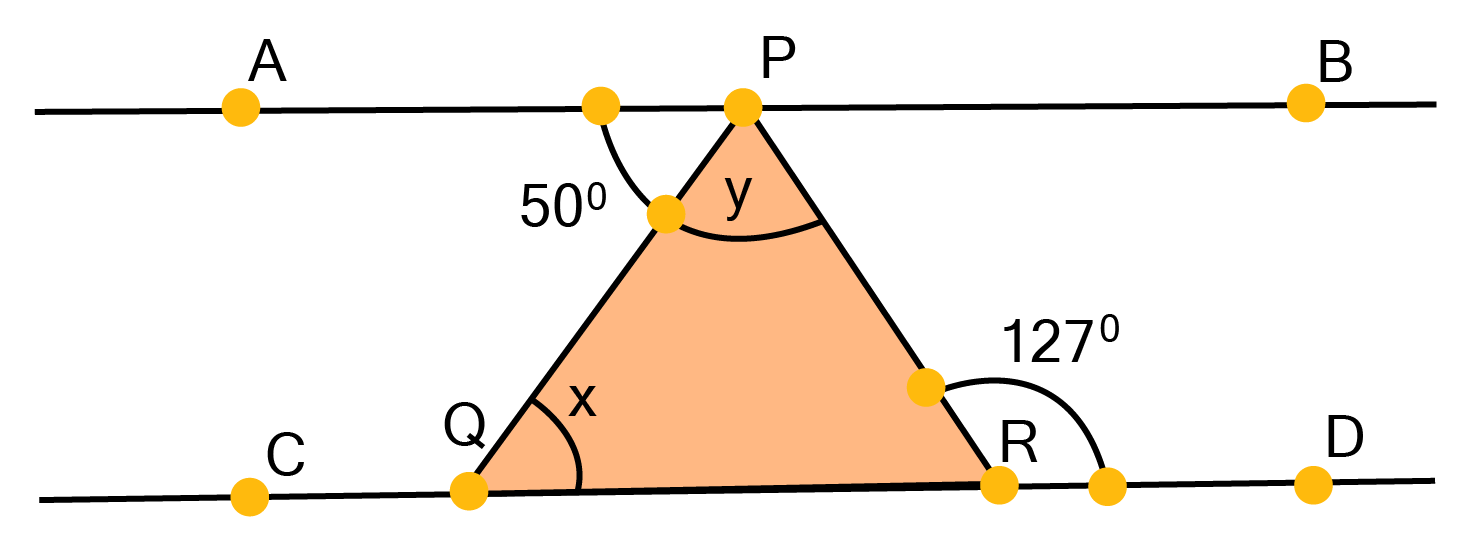Ans:

$\angle APR=PRD$ (since alternate interior angles are equal)

$\therefore \text{5}{{\text{0}}^{\circ }}+y={{127}^{\circ }}$ (since $\angle APR=\angle \text{APQ+}\angle \text{PQR}$)

$\Rightarrow \text{y=7}{{\text{7}}^{\circ }}$

similarly,

$\angle \text{APQ=PQR}$ ∠APQ = ∠PQR (since alternate interior angles are equal)

$\therefore \text{x=5}{{\text{0}}^{\circ }}$

Therefore we found that $\text{x=5}{{\text{0}}^{\circ }}$ and $\text{y=7}{{\text{7}}^{\circ }}$

### Question 6

In the given figure, PQ and RS are two mirrors placed parallel to each other. An incident ray AB strikes the mirror PQ at B, the reflected ray moves along the path BC and strikes the mirror RS atC and again reflects back along CD. Prove that AB||CD.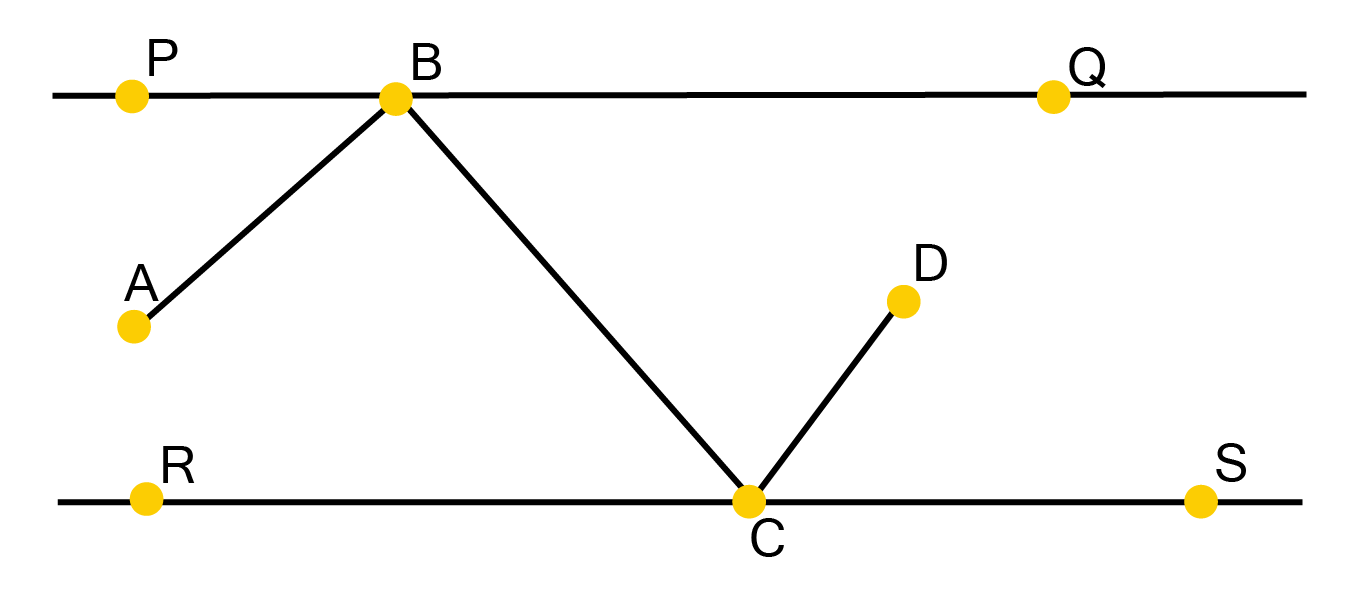Ans: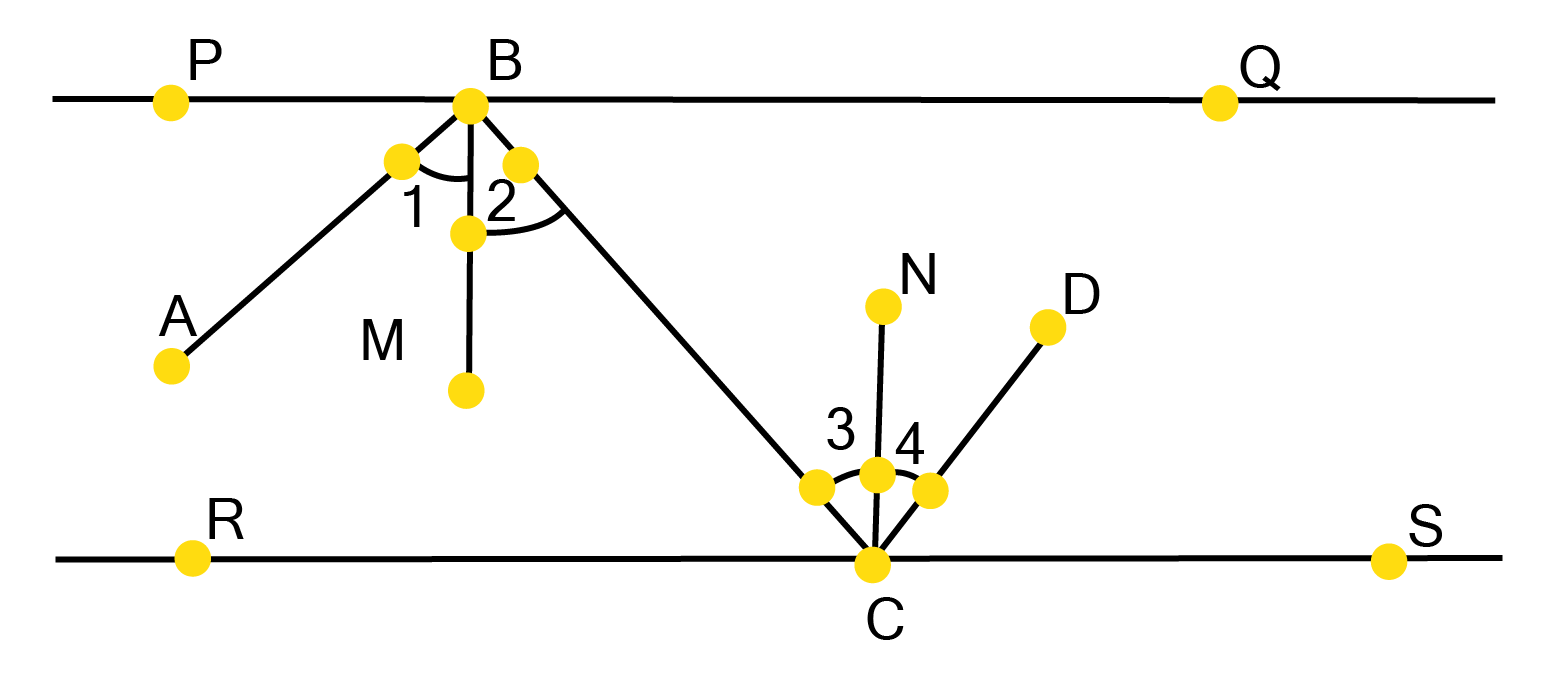Let us construct BM$\bot$ PQ and CN$\bot$RS.

Since  PQ$\parallel$ RS, and so BM $\parallel$ CN

Therefore, CN and BM are two parallel lines and a transversal line BC cuts them at B and C respectively.

∠2 = ∠3......($1$) (since alternate interior angles are equal)

But, by laws of reflection in Physics

∠1 = ∠2 and ∠3 = ∠4

Now from equation ($1$)

∠1 = ∠2 = ∠3 = ∠4

Therefore

∠1 + ∠2 = ∠3 + ∠4

$\angle \text{ABC=}\sum \text{DCB}$

But, these are alternate interior angles.

$\therefore$ AB$\parallel$ CD

Hence  proved.

### Question 1

In the given figure, sides QP and RQ of $\Delta$PQR are produced to points S and T respectively. If ∠SPR = 135o and ∠PQT = 110o, find ∠PRQ.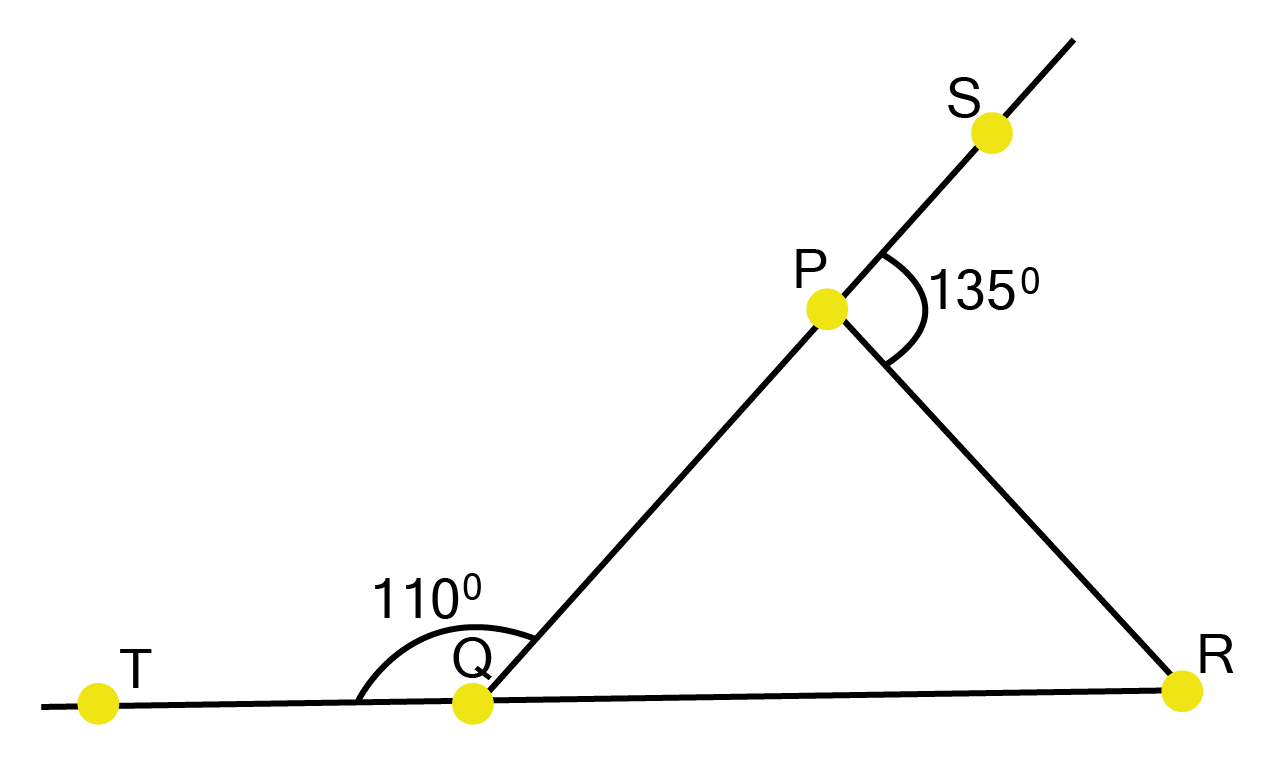Ans:

We are given that,

$\angle \text{SPR=13}{{\text{5}}^{\circ }}$ and$\angle \text{PQT=11}{{\text{0}}^{\circ }}$

Therefore we can write

$\text{ }\angle \text{SPR+}\angle \text{QPR=18}{{\text{0}}^{\circ }}$ (since Linear pair angles equals $\text{18}{{\text{0}}^{\circ }}$)

$\Rightarrow {{135}^{\circ }}+\angle \text{QPR=18}{{\text{0}}^{\circ }}$

$\therefore \text{QPR=4}{{\text{5}}^{\circ }}$

Similarly $\angle \text{PQT+PQR=18}{{\text{0}}^{\circ }}$ (Linear pair angles)

$\Rightarrow {{110}^{\circ }}+\angle PQR={{180}^{\circ }}$

From here we get

$\angle \text{PQR=7}{{\text{0}}^{\circ }}$

Considering $\Delta$ PQR,

$\angle \text{QPR+}\angle \text{PQR+}\angle \text{PRQ}={{180}^{\circ }}$ (sum of all interior angles of a triangle is ${{180}^{\circ }}$)

$\Rightarrow {{45}^{\circ }}+{{70}^{\circ }}+\angle PRQ={{180}^{\circ }}$

Hence we found that

$\text{ }\angle \text{PRQ=6}{{\text{5}}^{\circ }}$

### Question 2

In the given figure,$\angle \text{X=6}{{\text{2}}^{\circ }}$, $\angle \text{XYZ=5}{{\text{4}}^{\circ }}$. If YO and ZO are the bisectors of $\angle \text{XYZ}$ and $\angle \text{XZY}$respectively of $\Delta$XYZ, find $\angle \text{OZY}$ and$\angle \text{YOZ}$.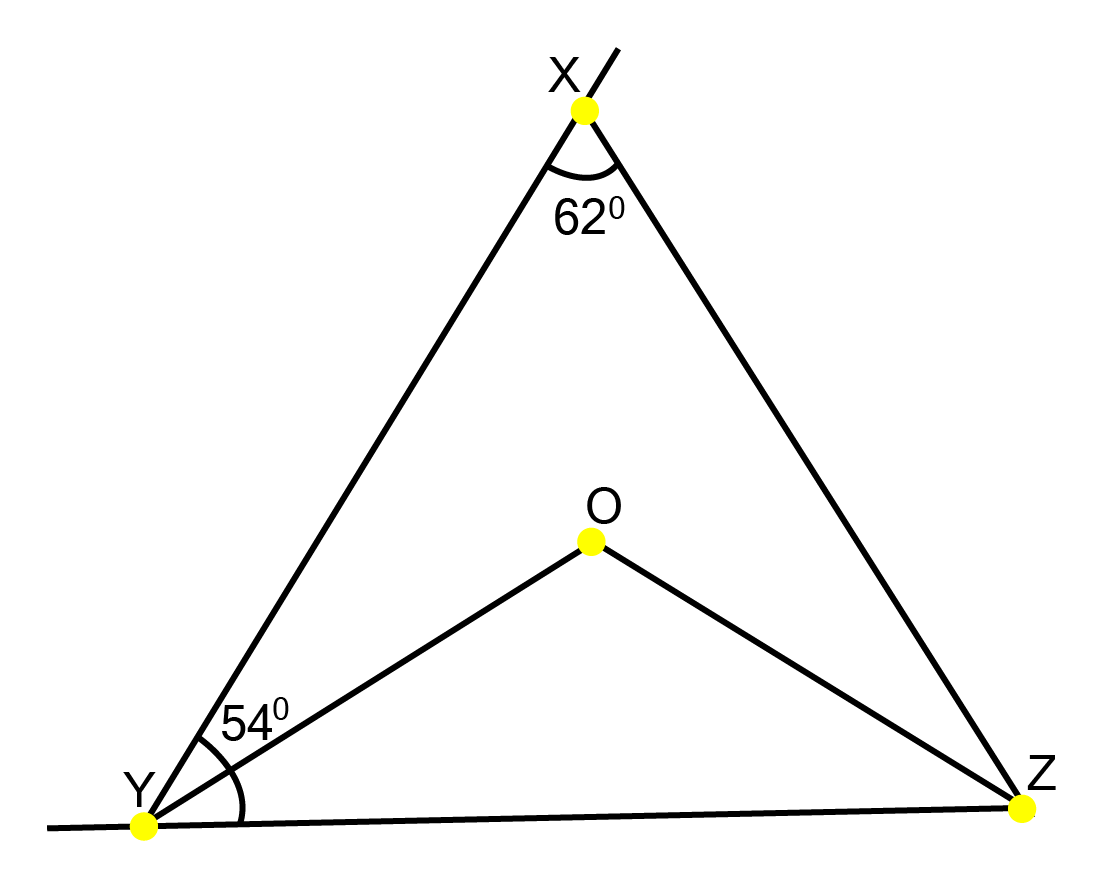Ans:

We know that  the sum of all interior angles of a triangle is${{180}^{\circ }}$, therefore, for $\Delta$ XYZ,

$\angle \text{X+}\angle \text{XYZ+XZY}$

$\Rightarrow {{62}^{\circ }}+{{54}^{\circ }}+\angle \text{XZY=18}{{\text{0}}^{\circ }}$

Hence we get

$\angle \text{XZY=6}{{\text{4}}^{\circ }}$

Similarly $\angle OZY={{27}^{\circ }}$ (since$\angle OZY=$$\frac{54}{2}$)

From $\Delta \text{OYZ}$ we obtain

$\angle OZY+\angle YOZ+\angle OZY=180$ ?(using angle sum property of a triangle)

$\Rightarrow {{27}^{\circ }}+\angle YOZ+{{32}^{\circ }}={{180}^{\circ }}$

From here we get

$\angle YOZ={{121}^{\circ }}$

Hence we found that, $\angle OZY={{27}^{\circ }}$and $\angle YOZ={{121}^{\circ }}$

### Question 3

In the given figure, if AB $\parallel$  DE, $\angle \text{BAC=3}{{\text{5}}^{\circ }}$ and$\angle \text{CDE=5}{{\text{3}}^{\circ }}$ , find $\angle DCE$.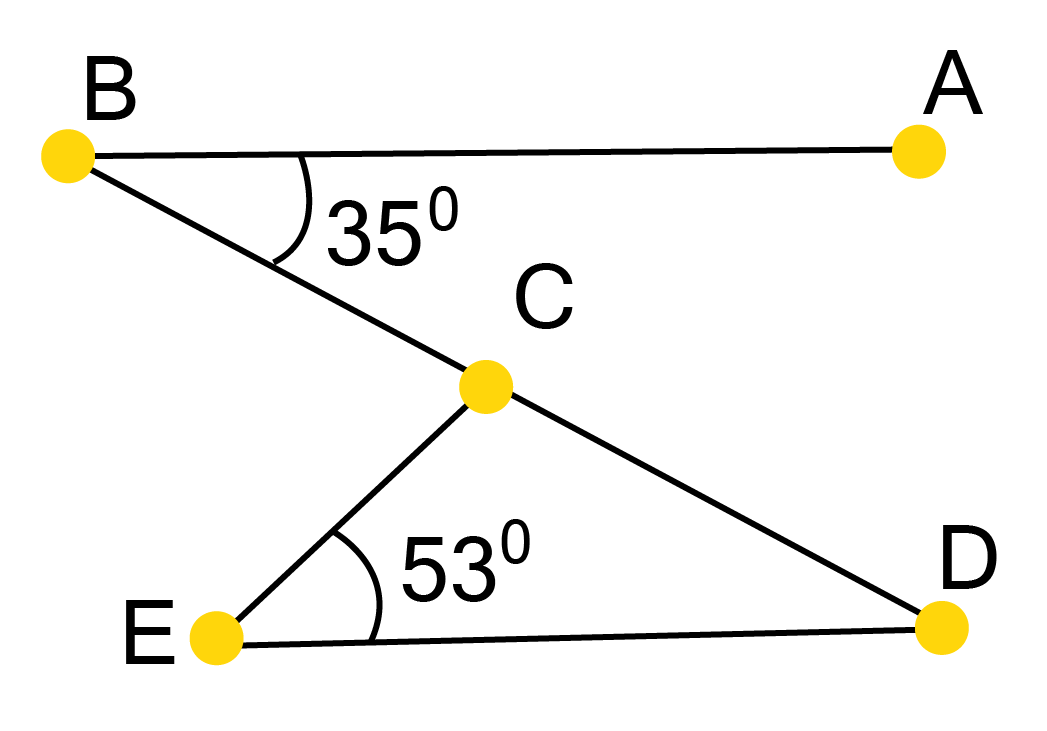Ans:

From the figure we can easily see AB $\parallel$  DE and AE is a transversal.

$\angle BAC=\angle CED$ (since alternate interior angles are equal)

$\angle \text{CED=3}{{\text{5}}^{\circ }}$

In $\Delta$CDE,

$\angle \text{CDE+}\angle \text{CED+}\angle \text{DCE=18}{{\text{0}}^{\circ }}$  (from the angle sum property of a triangle)

$\text{5}{{\text{3}}^{\circ }}+{{35}^{\circ }}+\angle DCE={{180}^{\circ }}$

From here we get

$\angle DCE={{92}^{\circ }}$

### Question 4

In the given figure, if lines PQ and RS intersect at point T, such that $\angle \text{PRT=4}{{\text{0}}^{\circ }}$,$\angle RPT={{95}^{\circ }}$and $\angle \text{TSQ=7}{{\text{5}}^{\circ }}$, find $\angle \text{STQ}$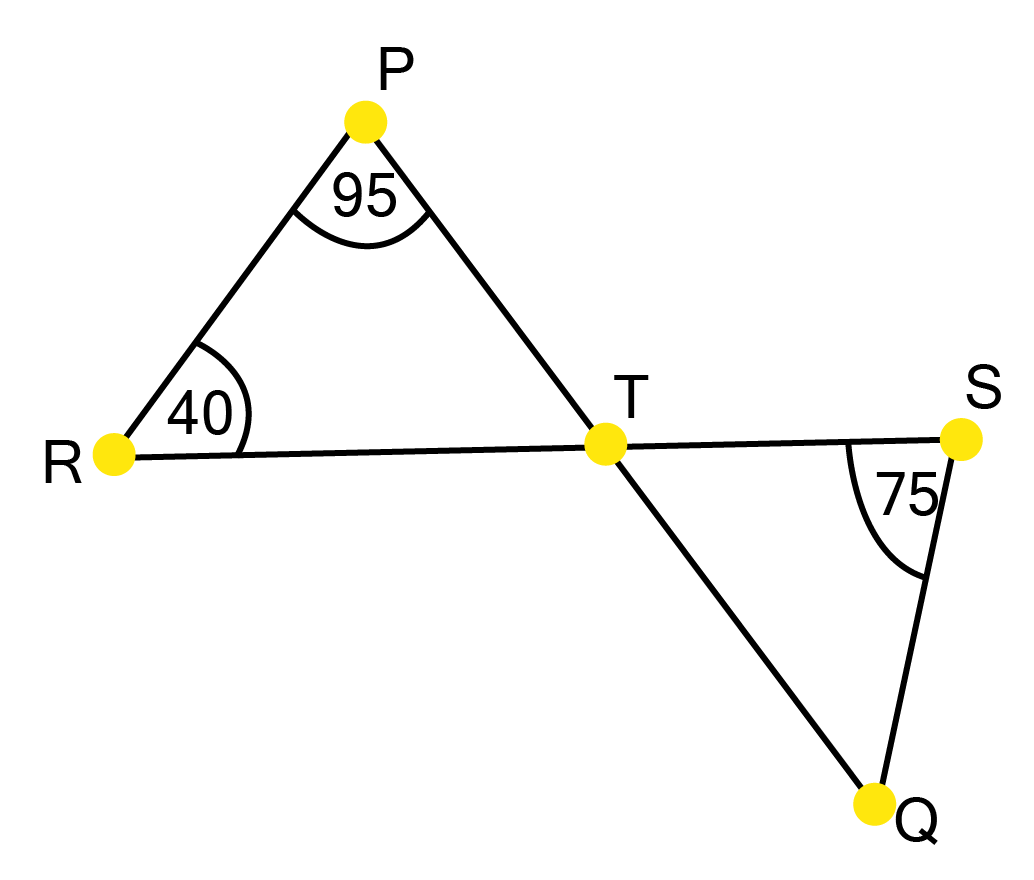Ans:

On applying angle sum property for $\Delta$PRT, we find

$\angle \text{PRT+}\angle \text{RPT+}\angle \text{PTR=18}{{\text{0}}^{\circ }}$

$\Rightarrow$ $\text{4}{{\text{0}}^{\circ }}+{{95}^{\circ }}+\angle PTR={{180}^{\circ }}$

From here we get

$\angle \text{PTR=4}{{\text{5}}^{\circ }}$

Now in $\Delta \text{STQ}$

$\angle STQ=\angle PTR$  (since they are vertically opposite angles)

$\therefore \angle STQ={{45}^{\circ }}$

Similarly on applying angle sum property for $\Delta$STQ, we find

$\angle STQ\text{+}\angle \text{SQT+}\angle \text{QST=18}{{\text{0}}^{\circ }}$

$\Rightarrow \text{ }{{45}^{\circ }}+\angle STQ+{{75}^{\circ }}={{180}^{\circ }}$

Hence we found that

$\angle \text{SQT=6}{{\text{0}}^{\circ }}$

### Question 5

In the given figure, if PQ $\bot$ PS, PQ $\parallel$ SR, $\angle \text{SQR=2}{{\text{8}}^{\circ }}$ and  $\angle \text{QRT=6}{{\text{5}}^{\circ }}$ then find the values of x and y.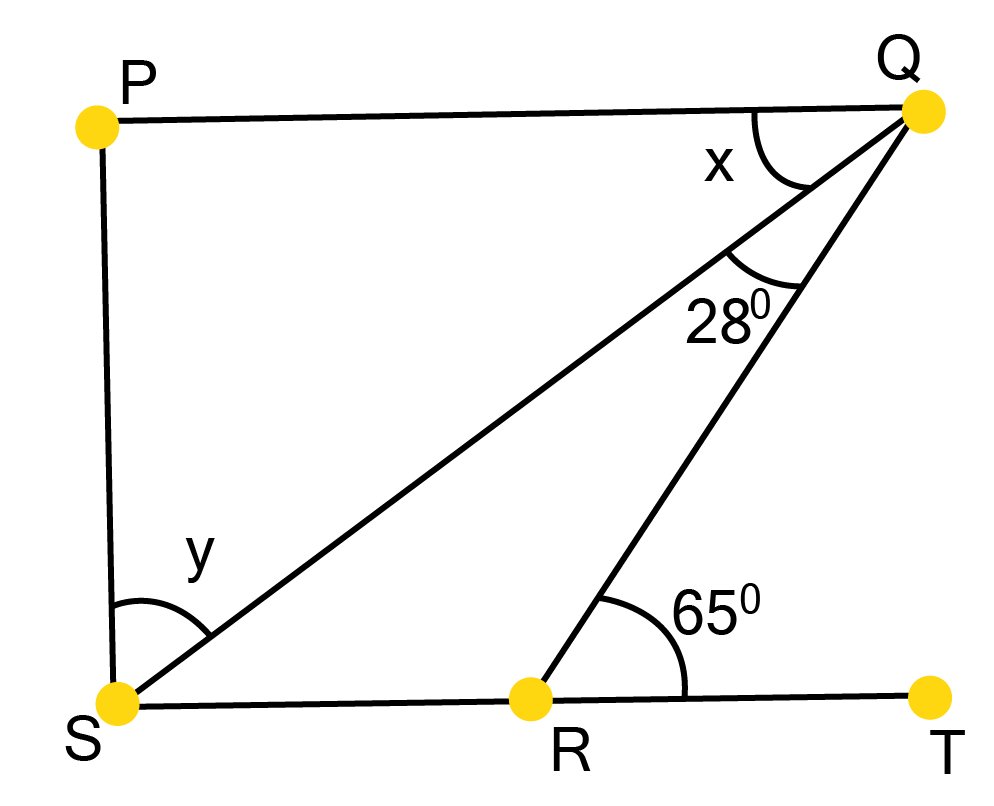Ans:

We are given that PQ$\parallel$ SR and QR is a transversal line.

$\therefore \angle \text{PQR=}\angle \text{QRT}$ (since alternate interior angles are equal)

$\Rightarrow x+{{28}^{\circ }}={{65}^{\circ }}$ (since $\angle \text{PQR=}\angle PQS+\angle SQR$)

From here we get

$\text{x=3}{{\text{7}}^{\circ }}$

By applying the angle sum property for $\Delta$SPQ, we find

$\angle SPQ+x+y={{180}^{\circ }}$

${{90}^{\circ }}+{{37}^{\circ }}+y={{180}^{\circ }}$

From here we get

$\text{y=5}{{\text{3}}^{\circ }}$

Hence we found and $\text{x=3}{{\text{7}}^{\circ }}$and $\text{y=5}{{\text{3}}^{\circ }}$

### Question 6

In the given figure, the side QR of $\Delta$PQR is produced to a point S. If the bisectors of $\angle PQR$ and $\angle \text{PRS}$ meet at point T, then prove that$\angle \text{QTR=}\frac{1}{2}\angle QPR$.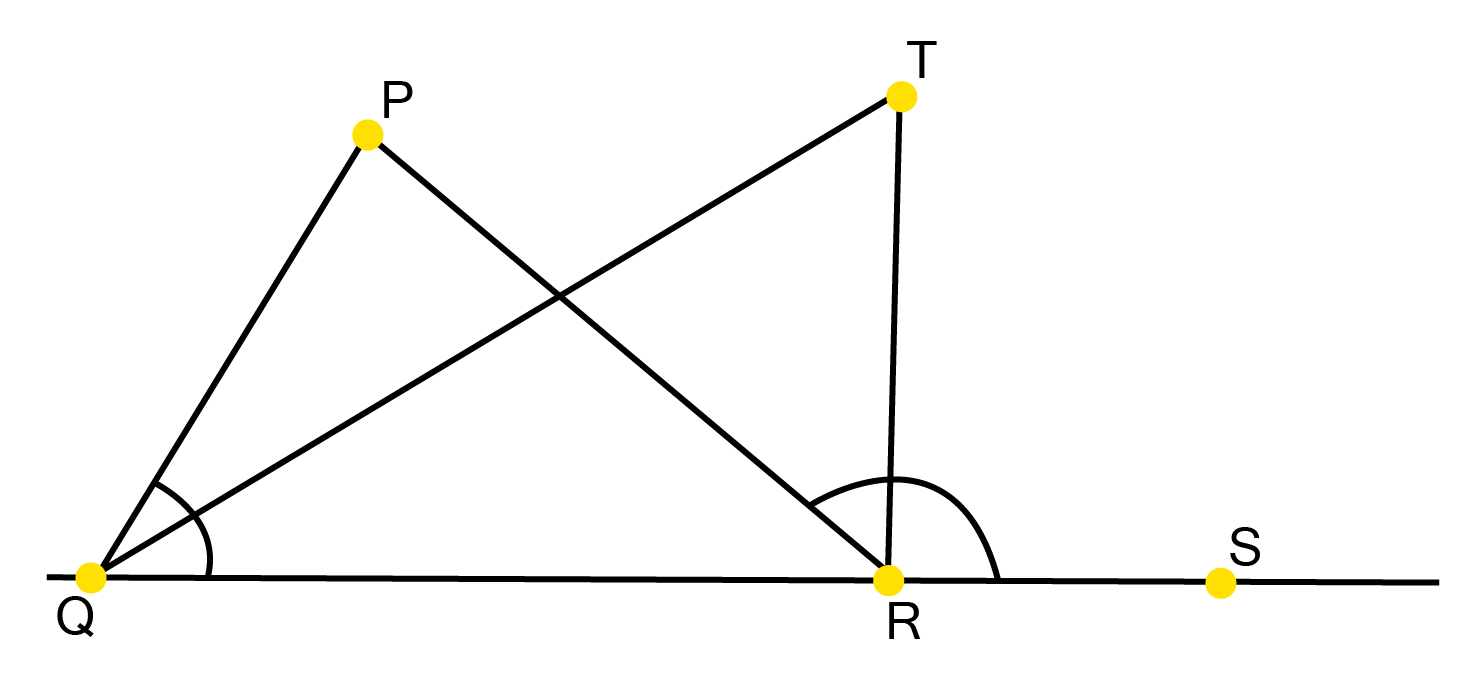Ans:

In $\Delta QTR$,we can easily observe ∠TRS is an exterior angle.

$\therefore \angle \text{QTR+}\angle \text{TQR=}\angle \text{TRS}$

$\Rightarrow \text{ }\angle \text{QTR=}\angle \text{TRS-}\angle \text{TQR}$...... ($1$)

In  $\Delta$PQR, we can easily observe $\angle \text{PRS}$ is an external angle.

$\therefore \angle QPR+\angle PQR=\angle PRS$

$\Rightarrow \angle QPR+2\angle TQR=2\angle TRS$ (Given QT and RT are angle bisectors) $\Rightarrow \text{ }\angle \text{QPR=2}\left( \angle TRS\text{-}\angle \text{TQR} \right)$

$\angle QPR=2\angle QTR$ (from equation $1$ )

Hence $\frac{1}{2}\angle QPR=\angle QTR$ proved.

### NCERT Solutions for Class 9 Maths Chapter 6 Lines and Angles - PDF Download

NCERT Solutions for Class 9 Maths Chapter 6 - Lines and Angles is a valuable resource for students embarking on a journey through the fascinating world of geometry. In this chapter, students explore the fundamental concepts of lines, angles, and their relationships, laying the groundwork for more advanced geometric studies. This PDF download provides a comprehensive guide to understanding and mastering the principles of this chapter. With clear explanations, illustrative diagrams, and step-by-step solutions, it offers students a reliable tool to enhance their mathematical proficiency. Whether you're preparing for exams or seeking to strengthen your geometric knowledge, these NCERT Solutions serve as a bridge to geometric excellence.

You can opt for Chapter 6 - Lines and Angles NCERT Solutions for Class 9 Maths PDF for Upcoming Exams and also You can Find the Solutions of All the Maths Chapters below.

### Important Points to Remember: NCERT Solutions Class 9 Maths Chapter 6

1. Angles and Their Properties

If θ is the angle between two rays, then which kind of angle it forms can be calculated as,

 0°＜θ ＜90° Acute angle θ = 90° Right angle 90 ＜θ ＜180° Obtuse angle θ = 180° Straight angle 180° ＜θ ＜360° Reflex angle

1. Let x and y be two angles, then:

• If x + y = 90°, x and y are said to be complementary angles.

• If x + y = 180°, x and y are said to be supplementary angles.

• If x and y have a common vertex and a common arm, they are said to be adjacent angles provided their non-common arms are on another side of the common arm.

• If the sum of the two adjacent angles is 180°, x and y are said to be linear pairs of angles.

1. When two lines intersect with each other, four angles are formed. Angles facing each other are called vertically opposite angles and they are equal.

2. The sum of all three angles of a triangle is 180°.

NCERT Solutions for Class 9 Maths Chapter 6 exercises 6.1, 6.2, and 6.3 are available on Vedantu in PDF format and students can download them for free.

### NCERT Solution Class 9 Maths of Chapter 6 includes 3 exercises.

 Chapter 6 - Lines and Angles Exercises in PDF Format Exercise 6.1 6 Questions & Solutions (5 Short Answers, 1 Long Answer) Exercise 6.2 3 Questions & Solutions (3 Short Answers, 3 Long Answers) Exercise 6.3 6 Questions & Solutions (5 Short Answers, 1 Long Answer)

### NCERT Solutions For Class 9 Maths Chapter 6 Lines and Angles - Free PDF Download

Lines and Angles Class 9 Solutions are prepared by highly qualified and professional teachers at Vedantu. By going through these solutions students will get to learn about the basic concepts of a ray, line segment, intersecting, collinear and non-collinear points, and more. Apart from accurate solutions, students should go through all the formulas and the steps of solving the sums of this chapter. These NCERT Solutions help students enhance their mathematics problem-solving skills. These Maths Class 9 Chapter 6 Solutions provided are prepared as per the latest guidelines of CBSE so that students can secure good marks in the exam by following these solutions.

### NCERT Solutions Class 9 Maths Chapter 6 - Lines and Angles

Class 9th Maths Chapter 6 educates students about the difference between a line segment and a ray. A line with two endpoints refers to a line segment whereas a ray is a part of a line with one endpoint. Students will get to know that if three or more points lie on the same line, then they are referred to as collinear points. When two lines initiate from one point, then an angle is formed. Lines and angles have various real-life applications. From designing a building structure to finding the height of a tower or an aircraft’s location, angles are used everywhere.

Class 9 Maths NCERT Chapter 6 explains how ‘vertically opposite angles’ are formed and measure to be equal when two lines intersect each other. Students have to understand the proof of this theorem. A transversal intersects two or more parallel lines at diverse points. When a transversal intersects a pair of parallel lines, then different angles are formed, namely, alternate interior angles, exterior angles, corresponding angles, etc.

### Class 9 Maths Chapter 6 Lines and Angles - Weightage Marks

The total weightage of the Geometry unit in class 9 Maths is 22 marks, out of which, this chapter holds a weightage of 6 marks. The sections covered in class 9 Maths ch 6 are as follows.

Ex 6.1 Introduction.

Ex 6.2 Basic Terms.

Ex 6.3 Intersecting Lines and Non-Intersecting Lines.

Ex 6.4 Pairs of Angles.

Ex 6.5 Parallel Lines and a Transversal.

Ex 6.6 Lines Parallel to the Same Line are parallel and concepts.

Ex 6.7 Angle Sum Property in a Triangle.

NCERT Solutions Class 9 Maths Chapter 6 - Lines and Angles available on Vedantu will help students to understand these concepts easily.

## Benefits of Lines and Angles Chapter 6 NCERT Solutions

NCERT Solutions for Class 9 Maths Chapter 6, make a well-organized study material for students. It helps them have a clear idea of the lines and angles and related topics. The step-by-step solutions are prepared by professional experts having several years of experience. While studying and preparing for the final exams, these solutions play a vital role. Students can get the confidence to solve the exercise sums and get a grasp of the concepts. Some of the benefits of NCERT Class 9 Maths Chapter 6 Solutions are as follows.

• The solutions on Vedantu cover each and every topic of the chapter perfectly so that students get a correct approach for the exam preparation.

• NCERT Solutions for Class 9 Maths Ch 6 present all the question answers in an efficient format.

• The easy-to-understand language, along with a well-structured format of these solutions, help aspirants in clearing all their doubts from this chapter.

• These solutions are error-free and hence allow students to grasp the in-depth knowledge of ch 6.

• The students can schedule their preparation from NCERT solutions class 9 Maths Chapter 6. With proper preparation, students can secure a good percentage.

## Conclusion

NCERT Solutions for Class 9 Maths Chapter 6 - Lines and Angles provide a comprehensive foundation in the fundamental principles of geometry. This chapter equips students with essential concepts, including definitions of lines, angles, and their classifications. It explores the properties of parallel lines, perpendicular lines, and transversals, offering a solid understanding of angle relationships. The chapter also introduces significant theorems, such as the Angle Sum Property of a Triangle and the Parallel Lines Converse Theorem, fostering problem-solving skills. Practical applications of these geometric principles are highlighted. Overall, this chapter empowers students to navigate the intricacies of geometry, paving the way for their continued mathematical journey.

## FAQs on NCERT Solutions for Class 9 Maths Chapter 6 - Lines And Angles

1. How can NCERT Solutions for Class 9 Chapter 6 Maths Help in the Exam Preparation?

NCERT Solutions for Class 9 Chapter 6 make comprehensive study material and cover the concepts of the entire chapter to help students build a strong foundation. These solutions follow the latest CBSE curriculum and make students familiar with the types of questions being asked from the lines and angles chapter. Students can get in-depth knowledge about the topic, brief summary, solved questions, and more. Apart from reasoning and logical skills, these chapter solutions offer comprehensive learning to students. The difficult topics like types of angles, interior, and exterior angles are explained in a better way. Students can clear their tough concepts and can analyze their weak areas with the guidance of NCERT solutions class 6.

2. How are the Vertically Opposite Angles Equal when Two Lines Intersect each Other?

Let AB and CD be two lines intersecting at a single point, say, P. APC and BPD, and APD and BOC form two pairs of vertically opposite angles. Additionally, ray AP is perpendicular on line CD. It implies the sum of APC and APD is equal to 180o. Now, the sum of angles APD and BPD is equal to 180o. By using these two equations, it’s clear that the sum of angles APC and APD is equal to the sum of APD and BPD angles. It implies angle APC is equal to BPD. Similarly, it can be shown that angle APD is equal to angle BPC.

3. Are all the exercises important in Chapter 6 of Class 9 Maths?

All exercises of NCERT Class 9 Maths Chapter 6 are designed in a well-structured hierarchy of difficulty to test the student’s ability in problem-solving. They also help him or her bridge the knowledge gap. Practising all the exercises is of utmost importance to score well and to clearly understand all the necessary concepts. Hence it is advisable to not skip any exercise. All the solutions are also easily available on Vedantu website and the app.

4. What is the best Solution book for Chapter 6 of NCERT Class 9 Maths?

Vedantu is the place where you will get the solutions easily and for free. To download the solutions for Chapter 6 of NCERT Class 9 Maths, follow the steps mentioned below:

1. Visit the page of Chapter 6 of NCERT Solutions for Class 9 Maths.

2. The website for solutions for Chapter 6 of Class 9 Maths will open.

3. The solutions for the selected chapter will be displayed on the screen. One can also download these solutions by clicking on Download PDF to save it offline.

For more information regarding this, you can visit the Vedantu site or app and avail of their services to crack your entrances and perform well in all of your exams.

5. What do you mean by collinear and non-collinear points?

The collection of three or more points that occur on the same straight line is known as collinear points. On multiple planes, collinear points may occur, but not on different lines. Collinearity is the property of points being collinear. As a result, any three or more points are only collinear if they are in the same straight line. If any of the points are not on the same line, they are considered non-collinear as a group. The size of the triangle formed by the three non-collinear points will always be greater than zero.

6. What are the types of lines in Chapter 6 of Class 9 Maths?

In Geometry, there are two types of lines: straight and curved. Straight lines are divided into two categories: horizontal and vertical. Parallel, intersecting, and perpendicular lines are examples of other types of lines. Knowledge of all these types and the formulae related to them are very crucial and hence you must practice them judiciously.

7. What do you mean by a vertex?

A vertex is a point in geometry where two or more curves, lines, or edges intersect. As a result of this definition, vertices can be described as the junction where two separate lines tend to meet and make an angle, as well as the corners of polygons and polyhedra. In mathematics, it is an angular corner formed by the intersection of two or more lines or edges between two faces. A vertex may be found in 2D shapes such as pentagons and squares, as well as 3D shapes such as pyramids and cuboids.